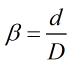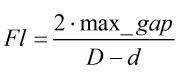# C029 – ISO 5167 – Orifice Plate Validation

## Description

This calculation is used to check the condition and geometry of an orifice plate meets the criteria laid out in the standard. The user can either enter measurements taken directly from an orifice plate or independently validate an orifice plate certificate produced and issued by a calibration laboratory.

ISO 5167-1:1991 – Measurement of fluid flow by means of pressure differential devices

ISO 5167-2:2003 – Measurement of fluid flow by means of pressure differential devices inserted in circular cross-section conduits running full- Part 2 Orifice Plates

Kelton calculation reference C029

FLOCALC calculation reference F071

## Options

### Standard

• 1991
• 2003

This option gives the choice of validating the orifice plate against either the 1991 or 2003 ISO 5167 standard.

### Flatness

• User entered
• Calculated from measured gap

This option allows the flatness to be user entered or calculated from a gap measured between the plate and a straight edge of length D laid across the diameter of the plate.

## Calculation

Note that most of the computations carried out by this calculation are taking the input data and validating that against conditions specified in ISO 5167. Due to this many of the useful outputs are to be found as warnings that will be displayed next to the relevant value. Here a statement will notify the user whether the criteria the orifice place must meet has been satisfied.

### Orifice Bore

The orifice bore is calculated by taking the mean of 4 measurements of the bore carried out at approximately equal angles to each other. Each of these diameters also must fulfil the validation criterion of deviating less than 0.05% from the calculated mean diameter.

### Beta Ratio

The beta ratio is calculated by:Where d = Mean orifice bore D = Meter tube bore

### Flatness

Flatness can be entered as a gradient or calculated from the gap between the orifice plate and a straight edge of length D laid across any diameter of the plate.

The flatness in the latter case is calculated by:Where max_gap = Maximum gap between orifice plate and a straight edge of length D laid across any diameter of the plate